Linear Transformations: Symmetry Operations on Crystal Structures

Group theory arises from the mathematics of relating the symmetry of objects.  In crystallography, it is applied to the classification of different types of arrangments of points (i.e. crystal lattices) and the symmetry operations include multiplication of matrices representing common symmetry operations. In this example, we will construct an FCC unit cell and then show that the cell remains invariant under different symmetry operations.  The set of all operations that leave a lattice invariant uniquely defines the space group of the lattice.  We build an FCC from graphics primiitves, and then translate it so the center is at the origin.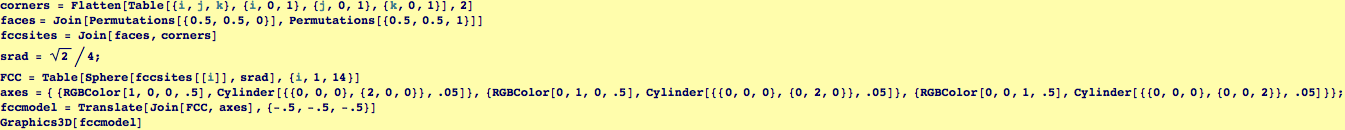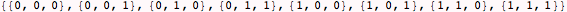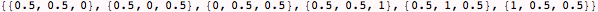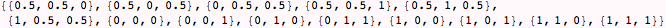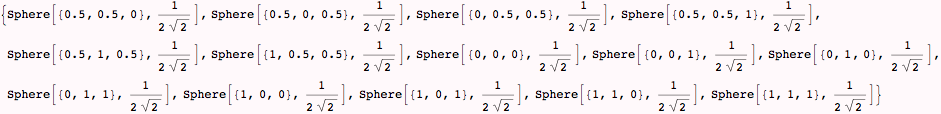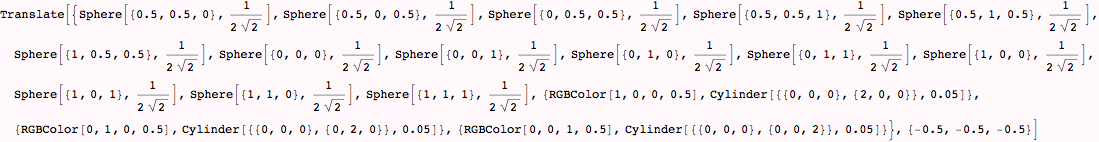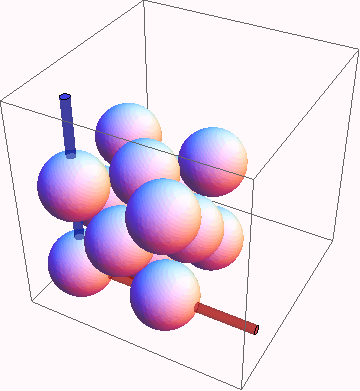We visualize the 3-fold rotation about , and the roto-inversion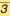(an inversion of all axis through the center, followed by a 3-fold rotation)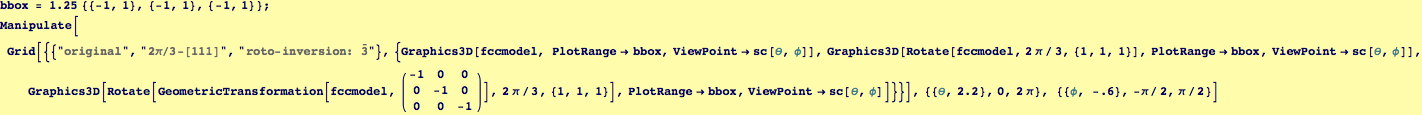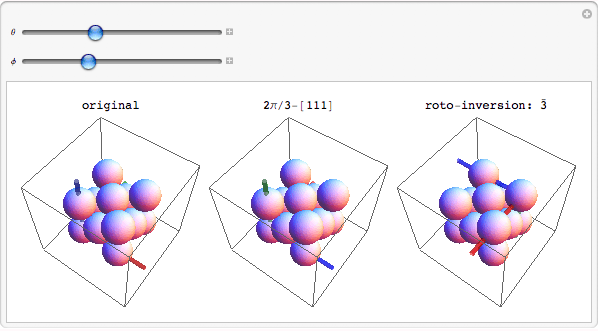Created by Wolfram Mathematica 6.0  (06 September 2007)